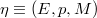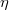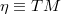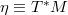ď»ż

### Introduzione

#### Abstract

En
The tangent and cotangent spaces of a bundle$\eta \equiv (E,p,M)$ are considered, analysing their affine structure on the horizontal and the vertical spaces,respectively. When the bundle$\eta$ is affine or linear, further structures are considered. These results are specialised for$\eta \equiv TM$ or$\eta \equiv T^*M$, finding interesting endomorphisms and isomorphisms. The Lie derivatives of tensors and the connections on bundle are introduced and analysed in strict relation with the affine structure of the tangent bundles.

DOI Code: §

Full Text: PDF